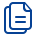﻿ 표지이미지 및 상세보기도서상세정보
DETAIL INFORMATIONFundamentals of Finite Element Analysis  : Linear Finite Element Analysis
독자층 : Professional & Vocational; Further/Higher Education
저      자:  Ioannis KoutromanosAuthor
출 판 사:  Wiley
출 판 일:  20180221
가      격:  \$ 120
I S B N:  9781119260080
페 이 지:  712 p.
판      형:  6.7 x 1.4 x 9.6 inches
커      버:  Paperback
An introductory textbook covering the fundamentals of linear finite element analysis (FEA)  This book constitutes the first volume in a two-volume set that introduces readers to the theoretical foundations and the implementation of the finite element method (FEM). The first volume focuses on the use of the method for linear problems. A general procedure is presented for the finite element analysis (FEA) of a physical problem, where the goal is to specify the values of a field function. First, the strong form of the problem (governing differential equations and boundary conditions) is formulated. Subsequently, a weak form of the governing equations is established. Finally, a finite element approximation is introduced, transforming the weak form into a system of equations where the only unknowns are nodal values of the field function. The procedure is applied to one-dimensional elasticity and heat conduction, multi-dimensional steady-state scalar field problems (heat conduction, chemical diffusion, flow in porous media), multi-dimensional elasticity and structural mechanics (beams/shells), as well as time-dependent (dynamic) scalar field problems, elastodynamics and structural dynamics. Important concepts for finite element computations, such as isoparametric elements for multi-dimensional analysis and Gaussian quadrature for numerical evaluation of integrals, are presented and explained. Practical aspects of FEA and advanced topics, such as reduced integration procedures, mixed finite elements and verification and validation of the FEM are also discussed.  Provides detailed derivations of finite element equations for a variety of problems. Incorporates quantitative examples on one-dimensional and multi-dimensional FEA. Provides an overview of multi-dimensional linear elasticity (definition of stress and strain tensors, coordinate transformation rules, stress-strain relation and material symmetry) before presenting the pertinent FEA procedures. Discusses practical and advanced aspects of FEA, such as treatment of constraints, locking, reduced integration, hourglass control, and multi-field (mixed) formulations. Includes chapters on transient (step-by-step) solution schemes for time-dependent scalar field problems and elastodynamics/structural dynamics. Contains a chapter dedicated to verification and validation for the FEM and another chapter dedicated to solution of linear systems of equations and to introductory notions of parallel computing. Includes appendices with a review of matrix algebra and overview of matrix analysis of discrete systems. Accompanied by a website hosting an open-source finite element program for linear elasticity and heat conduction, together with a user tutorial. Fundamentals of Finite Element Analysis: Linear Finite Element Analysis is an ideal text for undergraduate and graduate students in civil, aerospace and mechanical engineering, finite element software vendors, as well as practicing engineers and anybody with an interest in linear finite element analysis.